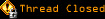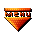# Topic awaiting preservation: convert string into number question! (Page 1 of 1)Hiroki
Paranoid (IV) Inmate

From: NZ
Insane since: Dec 2002posted 05-29-2003 23:32

Hi, guys. Good morning. How are you?
I probably failed database test yesterday.
Never mind. I will do resit.

Well, I have got an important question about strings and numbers.

quote:
If the user types a number in a text box, JavaScript stores it as a string, but to work with it as a number, you'll need to convert it.

This is said in my book from library.
But honestly I haven't done any converting so far to calculate numbers.

code:
```<html>
<script type="text/javascript">
function cal()
{
var one=document.theForm.text1.value
var two=document.theForm.text2.value
var prod=one*two
alert("the result of " + one + " by " +two + " is " + prod +" . ")
}
</script>
<body>
<form name="theForm">
<p>
<input type="text" name="text1" size="5">
by
<input type="text" name="text2" size="5">

</p>
<input type="button" value="Calculate!" onclick="cal()">
<input type="reset" value="Reset">
</form>
</body>
</html>```

This code is working fine. Here is uploading file.

Originally example code was like below:

code:
```function doMoth()
{
var one = eval(document.theForm.elements.value)
var two = eval(document.theForm.elements.value)
var prod = one*two
alert("The procuct of " +one + "and " + two + " is " + prod + ".")
}```

I could not understand eval so that just deleted like above.
Both of them worked fine.

But today, I got an serious problem.
I coded simple adding calculator without converting string to numbers.
As you expect It didn't work like I wanted.

In my guess, anyway must convert string into number when I do math. Because JavaScript treat all input text data as string not numbers.

Is that right????

Hiroki Kozai

Dracusis
Maniac (V) Inmate

From: Brisbane, Australia
Insane since: Apr 2001posted 05-30-2003 00:35

eval() is a hard one to come to terms with... It will evaluate a string as if it's contents were JavaScript code. In other words, if you have a string value that can be executed as JavaScript code, it will execute it.

So if you did this:

stringValue = "document.write('Boo!')"

eval(stringValue)

It would write Boo! to the document. Thus if you eval a string like "72" it would treated it as the number 72. Another simple and less resource intensive way to do this is to use the parseInt() function like so:

var one = parseInt(document.theForm.text1.value)

In any event, your code works fine so I wouldn't worry too much about it . Or did I not understand the question... You said you had problems but you've linked to some code that works fine??? If you have a problem, post the code that actually has the problem. Anyways, with the example you linked to if I enter 12px by 2px your calculator returns the result as NaN (Not a Number). If you were using parseInt() it would effectively chop any a-z characters off the end, parseInt() is also handy when dealing with pesky npx values from CSS.

Hiroki
Paranoid (IV) Inmate

From: NZ
Insane since: Dec 2002posted 05-30-2003 01:20

Hi, Dracusis.
How are you today?
I guess it is about 10 past 9 there, A?
Would you see my problem here.

When I input number, it is teated as string. So answer is like 2 + 2 = 22.
I understand why this happens. Because input text is treated as string not number, right? That is why I need to put parseInt or eval in front of document.num.value.

But what I am confused is.....Please see this code below:

code:
```function cal()
{
var one=document.theForm.text1.value
var two=document.theForm.text2.value
var prod=one*two   alert("the result of " + one + " by " +two + " is " + prod +" . ")
}
</script>```

In this code, I didn't do like eval(document.theForm.text.value). But it worked fine!
Why don't I have to put eval???? My understanding is that when I do math using Javascript, must convert string to number otherwise doesn't work properly.
But I found very confusing exception like above.

Hiroki Kozai

Slime
Lunatic (VI) Mad Scientist

From: Massachusetts, USA
Insane since: Mar 2000posted 05-30-2003 05:03

Ah, the thing about JavaScript is that you don't *have* to do the conversion yourself. When JavaScript sees that you're trying to multiply two strings together, it realizes that it can't multiply strings, so it *automatically* converts the strings into numbers for you.

The reason you *want* to convert the numbers yourself is that it won't always happen automatically. For instance, if you try to add the two numbers together, the browser will first try to add the *strings* together.

So this:

"2" + "2"

results in

"22"

So that's a case where you want to do the conversion yourself. The reason multiplication works is that strings can't be multiplied, so the browser has no choice but to automatically convert to numbers. If you tried this:

you would get the special value called NaN, which is short for Not a Number. It tries to convert the strings into numbers, but the strings don't contain any digits, so they get converted into the special NaN value. NaN * NaN = NaN. (In fact, NaN mixed with another number in any way always gives back NaN.) NaN is generally something you want to avoid.

The automatic conversion is convenient sometimes, but you shouldn't rely on it, because that can lead to bugs that are hard to figure out.

Hiroki
Paranoid (IV) Inmate

From: NZ
Insane since: Dec 2002posted 05-30-2003 05:22

Hi, Slime.
I understand that.
Many many thank you for that.
Now I am MA610 class, which is about Flash scripting.
Cya.

Hiroki Kozai